# Installing EPICS Core System and CSS Studio on Red Hat Linux— Install EPICS BASE —

Become superuser

Setup HTTP and HTTPS proxy (If you are not using one - just omit these lines)

Make EPICS base directory

Set EPICS CONFIG parameters (These parameters are for 64 bit version)

Install readline library and run ‘make’

— Install CSS Studio —

Unzip files and change owner of CSS folder to ‘user’

# How to Model ADC Quantization Effect With Known Maximum Voltage and Number of Bits and Apply It to a Signal in Matlab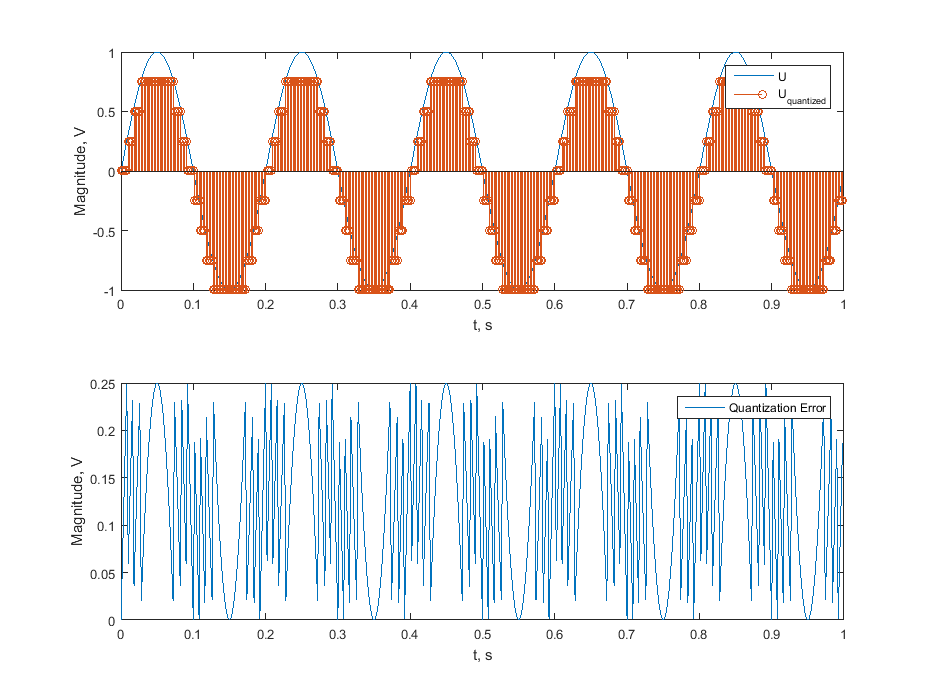# Python Script to Download Gost From protect.gost.ru as a Pdf File

Unfortunately the Russian database of the national standards (GOSTs) does not enable to get files as .pdf , but only as separate images (which are even not directly available). So we can’t even download the texts, it’s a pity. I’ve made a simple Python script to address this problem, because it’s far better to read and explore a printed copy. Script uses some libraries, you can install them by running pip install img2pdf pypdf2 requests It was tested in Python 2.7.Usage:

2. Get the link, in the example it is http://protect.gost.ru/v.aspx?control=8&baseC=-1&page=0&month=-1&year=-1&search=&RegNum=1&DocOnPageCount=15&id=126445
3. Replace the link in the script
4. Run the script python gostdl.py
5. In the same folder you will get separate pages of document in .pdf and .jpg format as well as merged document in document.pdf

# Fixing Windows From BSOD (STOP 7B 0x0000007B) After a Motherboard Replacement

After the motherboard replacement I’ve got a blue screen of death (BSOD). This can happen after hard disk replacement also. I’ve used the recipe from here to recover from this failure.

2. Put the script file into the root of your USB Flash
3. Boot to the Recovery Console (F8 > Repair Your Computer) or from a system repair/Windows 7 disc.
4. Launch the command prompt.
5. To determine the name of the USB Flash disk you can use wmic logicaldisk get name,description
6. Change directory to the root of USB disk, e.g cd E:\
7. Run the script cscript fix_7hdc.vbs /enable /search

# How to Effectively Convert Books From DJVU to PDF

DJVU is a very good, although not very portable format for books and documents. PDF is less efficient in compression, but is far more popular and portable. You can easily find a free PDF reader for any system, for example for iPhone or iPad, but opening and reading DJVU is harder.

Here are the instructions:

1 - Install DJVULibre. It is available for Windows, Linux and MacOS.

2 - Use File -> Export As…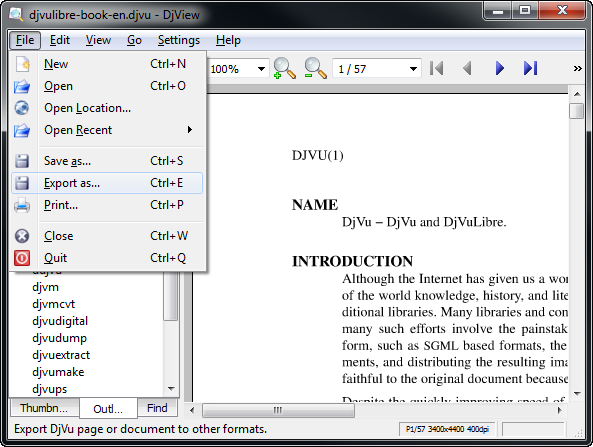3 - Choose export settings4 - Choose compression settings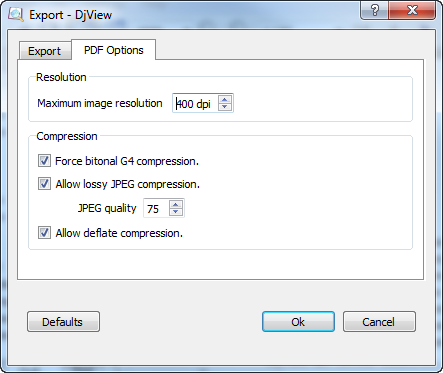5 - You can also convert books from the command line (good for batch procesing)

# Calculate Powers in Non-sinusoidal Conditions According to IEEE 1459

Definitions of electric power qualities under nonsinusoidal conditions are quite complex and hard to grasp. Annex B of the IEEE Std 1459-2010 shows how to calculate apparent power components in non-sinusoidal conditions. I have written a MATLAB script to illustrate this example. Unfortunately, there were typo errors in the standard, that’s why some of the values are different in my example.

Figure 1 — Instantaneous voltage and current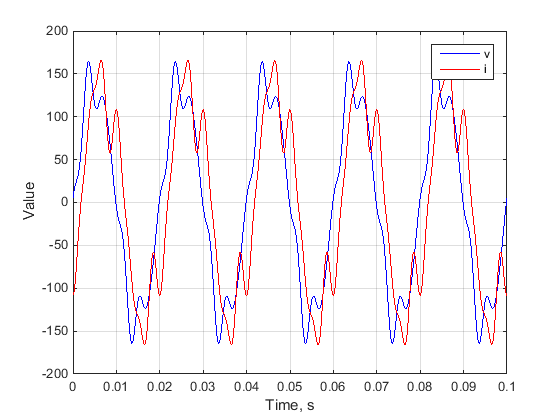A load is supplied with a nonsinusoidal voltage

$v=v_1 + v_3 + v_5 +v_7 = \sqrt{2} \sum_{h=1,3,5,7}V_h \sin(h \omega t-\alpha_h)$

has a nonsinusoidal current

$i=i_1 + i_3 + i_5 +i_7 = \sqrt{2} \sum_{h=1,3,5,7}I_h \sin(h \omega t-\beta_h)$

(To simplify the explanations, the eventual presence of dc components was ignored.) In this case, the instantaneous power has 16 terms that can be separated in two groups

$p=vi=p_{hh}+p_{mn}$

where

$p_{hh}=v_1 i_1 + v_3 i_3 +v_5 i_5 +v_7 i_7 = \sum_{h=1,3,5,7} v_h i_h$

is the instantaneous power that contains only direct products (i.e., each component is the result of interaction of voltage and current harmonics of the same order).

$p_{mn}=v_1 (i_3 +i_5 +i_7) + v_3 (i_1 +i_5 +i_7) +v_5 (i_1 +i_3 +i_7) +v_7 (i_1+i_3+i_7) =$ $= \sum_{m=1,3,5,7} v_m \sum_{n =1,3,5,7 \atop n \neq m} i_n$

is the instantaneous power that contains only cross products (i.e., each component is the result of interaction of voltage and current harmonics of different orders).

The direct products yield

$v_h i_h=\sqrt{2} \, V_h \sin (h \omega t - \alpha_h) \sqrt{2} \, I_h \sin (h \omega t - \beta_h) =$ $= P_h [1 - \cos(2 h \omega t - 2 \alpha_h)] - Q_h \sin (2 h \omega t - 2 \alpha_h)$

where

$P_h = V_h I_h \cos (\theta_h)$ $Q_h =V_h I_h \sin (\theta_h)$

are the harmonic active and reactive powers of order $h$, respectively, and $\theta_h = \beta_h - \alpha_h$ is the phase angle between the phasors $V_h$ and $I_h$.

The total active power is

$P = \sum_{h=1,3,5,7} P_h =P_1 +P_H$

where

$P_1 = V_1 I_1 \cos(\theta_1)$

is the fundamental (power-frequency) active power, and

$P_H=P_3+P_5+P_7 = \sum_{h \neq 1} P_h$

is the total harmonic active power.

For each harmonic order, there is an apparent power of order h

$S_H=\sqrt{P_h^2 +Q_h^2}$

The cross-products of the instantaneous powers have expressions as follows:

$v_m i_n=\sqrt{2} \, V_m \sin (m \omega t - \alpha_m) \sqrt{2} \, I_n \sin (n \omega t - \beta_n) =$ $= D_{mn} \left( \cos[(m-n) \omega t - \alpha_m + \beta_n ] + \cos[(m+n) \omega t - \alpha_m - \beta_n] \right)$

where

$D_{mn}=V_m I_n$

The total apparent power squared

$S^2 = V^2 I^2 =(V_1^2 +V_3^2 +V_5^2 +V_7^2)(I_1^2 +I_3^2 +I_5^2 +I_7^2)$

may be separated in the same manner as the instantaneous power, in direct and the cross-products:

$S^2 = V_1^2 I_1^2 +V_3^2 I_3^2 + V_5^2 I_5^2 + V_7^2 I_7^2 +$ $+ V_1^2 (I_3^2 +I_5^2 +I_7^2) + I_1^2 (V_3^2 +V_5^2 +V_7^2)+$ $+ V_3^2 I_5^2 + V_3^2 I_7^2 + V_5^2 I_3^2 + V_5^2 I_7^2 + V_7^2 I_3^2 + V_7^2 I_5^2$

or

$S^2 = S_1^2 +S_3^2 +S_5^2 +S_7^2 +$ $+ D_I^2 +D_V^2 +$ $+ D_{35}^2+ D_{37}^2 + D_{53}^2 + D_{57}^2 + D_{73}^2 + D_{75}^2 = S_1^2 + S_N^2$

where

$S_1^2 = P_1^2 + Q_1^2$

with $S_1$ , $P_1$ and $Q_1$ are the apparent, active, and reactive fundamental powers, and

$S_N^2 = D_I^2 + D_V^2 +S_H^2$

where

$D_I^2 = V_1^2 (I_3^2 +I_5^2 +I_7^2)$

is the current distortion power,

$D_V^2 = I_1^2 (V_3^2 +V_5^2 +V_7^2)$

is the voltage distortion power, and

$S_H^2 = S_3^2 +S_5^2 +S_7^2 +$ $+ D_{35}^2+ D_{37}^2 + D_{53}^2 + D_{57}^2 + D_{73}^2 + D_{75}^2 =$ $= P_3^2 + P_5^2 + P_7^2 + Q_3^2 + Q_5^2 + Q_7^2 +$ $+ D_{35}^2+ D_{37}^2 + D_{53}^2 + D_{57}^2 + D_{73}^2 + D_{75}^2 \qquad (1)$

is the harmonic apparent power

If the load is supplied by a line with a resistance $r$ the power loss in the line is

$\Delta P = r I^2 = \frac{r}{V^2} S^2 = \frac{r}{V^2} (S_1^2 + S_N^2) =$ $= \frac{r}{V^2} (P_1^2 +Q_1^2 +D_I^2 +D_V^2 +S_H^2) \qquad (2)$

It is learned from this expression that every component of $S$ contributes to the total power loss in the supplying system. This means that not only fundamental active and reactive powers cause losses but also the current and voltage distortion powers as well as the harmonic apparent power cause losses.

The following numerical example is meant to facilitate the understanding of the previous explanations:

The instantaneous voltages and currents are

$\begin{array}{c c} v_1=\sqrt{2} \, 100 \sin (\omega t - 0^{\circ} ) & i_1=\sqrt{2} \, 100 \sin (\omega t - 30^{\circ} ) \\ v_3=\sqrt{2} \, 8 \sin (\omega t - 70^{\circ} ) & i_3=\sqrt{2} \, 20 \sin (3 \omega t - 165^{\circ} ) \\ v_5=\sqrt{2} \, 5 \sin (\omega t + 140^{\circ} ) & i_5=\sqrt{2} \, 15 \sin (5 \omega t + 233^{\circ} ) \\ v_7=\sqrt{2} \, 7 \sin (\omega t + 20^{\circ} ) & i_7 =\sqrt{2} \, 10 \sin (7 \omega t + 288^{\circ} ) \\ \end{array}$

The calculated active powers are summarized in Table 1.

Table 1 — Active powers

$\begin{array}{|c|c|c|c|c|c|} \hline P_1 (W) & P_3 (W) & P_5 (W) & P_7 (W) & P (W) & P_H (W) \\ \hline 8660.25 & -13.94 & -11.78 & -1.74 & 8632.79 & -27.47 \\ \hline \end{array}$

The total harmonic active power $P_H =P –27.47 W < 0$ is supplied by the load and injected into the power system. This condition is typical for dominant nonlinear loads. The bulk of the active power is supplied to the load by the fundamental component $P_1 = 8660.25 W$ .

The four reactive powers are given in Table 2.

Table 2 — Reactive powers

$\begin{array}{|c|c|c|c|} \hline Q_1 (var) & Q_3 (var) & Q_5 (var) & Q_7 (var) \\ \hline 5000.00 & 159.39 & -224.69 & 49.97 \\ \hline \end{array}$

Of interest is the fact that $Q_5 < 0$ , whereas other reactive powers are positive. If one incorrectly defines a total reactive power as the sum of the four reactive powers (in accordance with C. Budeanu’s definition):

$Q_B = Q_1 +Q_3 +Q_5 +Q_7 = 4984.67 \, var$

and assumes that the supplying line has a resistance $r= 1.0 \, \Omega$ and the load is supplied with an rms voltage $V=240 V$, the power loss due to $Q_B$ in line is

$\Delta P_B = \frac{r}{V^2}Q_B^2= \frac{1}{240^2}4984.67^2 = 431.37 \, W$

According to the previous analysis, [see Equation (1) and Equation (2)], the correct way to find the corresponding power loss due to $Q_1$ , $Q_3$ , $Q_4$ , $Q_7$ is

$\Delta P = \frac{r}{V^2}(Q_1^2 +Q_3^2 +Q_5^2 +Q_7^2)= 435.39 \, W > P_B$

The reactive power $Q_5$ , despite its negative value, contributes to the line losses in the same way as the positive reactive powers. The fact that harmonic reactive powers of different orders oscillate with different frequencies reinforces the conclusion that the reactive powers should not be added arithmetically (as recommended by Budeanu).

The cross-products that produce the distortion powers $D_I$ and $D_V$ are given in Table 3.

Table 3 — Distortion powers and their components

$\begin{array}{|c|c|c|c|} \hline D_{13} (var) & D_{15} (var) & D_{17} (var) & D_{I} (var) \\ \hline 2000.00 & 1500.00 & 1000.00 & 2692.58 \\ \hline D_{31} (var) & D_{51} (var) & D_{71} (var) & D_{V} (var) \\ \hline 800.00 & 1500.00 & 500.00 & 1772.00 \\ \hline \end{array}$

Finally the remaining cross-products that belong to the harmonic apparent power are presented in Table 4.

Table 4 — Distortion harmonic powers

$\begin{array}{|c|c|c|c|c|c|} \hline D_{35} (var) & D_{37} (var) & D_{53} (var) & D_{57} (var) & D_{73} (var) & D_{75} (var) \\ \hline 120.00 & 80.00 & 300.00 & 150.00 & 100.00 & 75.00 \\ \hline \end{array}$

The studied system has the rms voltage and current: $V= 101.56 \, V$ and $I= 103.56 \, A$ with the total harmonic distortions $THD_V = 0.177$ and $THD_I = 0.269$

The apparent power and its components are represented in the following tree:

Figure 2 — Apparent power and its components treeThe fundamental power factor (displacement power factor) is $PF_1 = P_1 / S_1 =0.866$, and the power factor is $PF= P/S=0.821$ . The dominant power components are $P_1$ and $Q_1$ . Due to relatively large distortion, $S_N$ is found to be a significant portion of $S$, and the current distortion power $D_I$ is the dominant component of $S_N$ .

Here is the MATLAB script that illustrates the previous material.

# How to Evaluate Voltage Unbalance Using Symmetrical Components Method, and Determine Magnitudes by Known K2U (K0U)

To evaluate voltage unbalance we can use Symmetrical components method proposed by Charles Fortescue. Vector of three phase voltages can be expressed as a sum of three vectors (phasors): zero- positive- and negative-sequence.

$U_{abc} = \begin{bmatrix} U_a \ U_b \ U_c \end{bmatrix} = \begin{bmatrix} U_{a,0} \ U_{b,0} \ U_{c,0} \end{bmatrix} + \begin{bmatrix} U_{a,1} \ U_{b,1} \ U_{c,1} \end{bmatrix} + \begin{bmatrix} U_{a,2} \ U_{b,2} \ U_{c,2} \end{bmatrix}$ $\alpha \equiv e^{\frac{2}{3}\pi i}$ \begin{align} U_{abc} &= \begin{bmatrix} U_0 \ U_0 \ U_0 \end{bmatrix} + \begin{bmatrix} U_1 \ \alpha^2 U_1 \ \alpha U_1 \end{bmatrix} + \begin{bmatrix} U_2 \ \alpha U_2 \ \alpha^2 U_2 \end{bmatrix} = \ \end{align} \begin{align} &= \textbf{A} U_{012} \end{align}

From these phasors we can derive negative-sequence voltage unbalance ratio K2U and zero-sequence voltage unbalance ratio K0U

$K_{2U} = \frac{U_2}{U_1}$ $K_{0U} = \frac{U_0}{U_1}$

Here is MATLAB script that produces the desired level of both negative- and -zero voltage unbalance ratio, then sets respective phasor magnitudes. After that we estimate unbalance using Symmetrical components method and draw a phasor diagramm.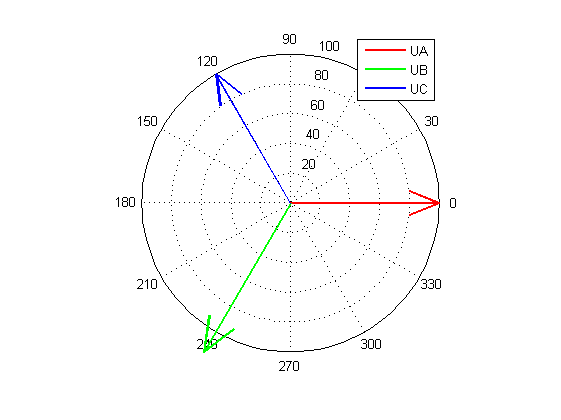To generate signal with known level of unbalance $K_{2U}$ we can change magnitude of one of the voltages in the balanced three phase system. So we set all angles between voltages = 120 deg. Magnitudes of Ua and Uc should be equal (Ua=Uc). Ub should be set as

$U_b = U_a \frac{1+2 K_{2U} }{1-K_{2U}}$

This equation can be easily derived if we notice that

$U_1 K_{2U} = \frac{1}{3} (U_b + 2 U_a) K_{2U} = \frac{1}{3} (U_b - U_a) = U_2$

# Drawing Horizontal and Vertical Lines in MATLAB Plot

Often we need to draw a vertical or horizontal line across the plot, to mark the boundaries or min/max value of the function. It’s not obvious at the first glance how to do it, becase the X and Y limits can be different, and also we need to handle zoom commands. I’m now using the solution, based on graph2d.constantline which was found here

It looks like this, and it also correctly handles zoom in and out commands.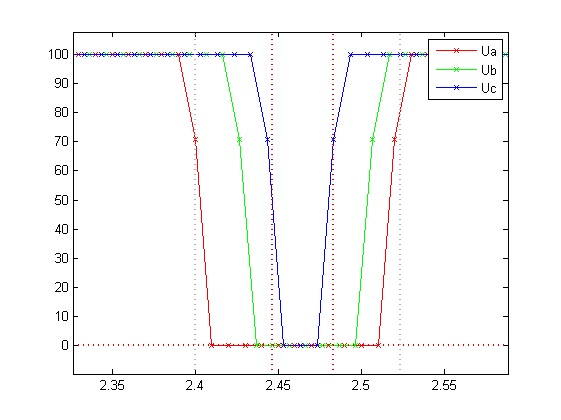UPD 03.04.2015 Unfortunately this does not work in Matlab 2014b or higher. Try this solution .

# Fast CSV Values Export to File. MEX-based Dlmwrite Replacement for MATLABMATLAB functions that export values into a .CSV file are very slow. It is said, that their inefficiency is rooted in bad implementation of the string concatenation. It is refered as Schlemiel the Painter’s algorithm.

Nevertheless, sometimes I have to write huge amounts of text data into CSV files. For example I had to generate test signals for power quality measurements algoritms, such as Flicker estimation. So I had written a MEX function to speed up this task. Write process can also be interrupted by pressing Ctrl-C using this wonderful trick (http://www.caam.rice.edu/~wy1/links/mex_ctrl_c_trick/).

Function takes four parameters

You can find the code and compiled mexw64 for function mex_WriteMatrix here

As you can notice difference in speed is huge! On big files ~ 1GB in size, write speed values are about 17-18 Megabytes/s.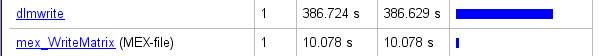You can use it freely, I hope it will save you a lot of time.

I use Coursera-dl to download lecture videos and materials from Coursera, so I can watch them later after the course is finished. How to install coursera-dl on Windows machine? It’s easy.2.Then install Pip (Python package manager)

• Run the command line cmd
• Change directory to your Python installation ( C:\Python27 for my case )
• execute python get-pip.py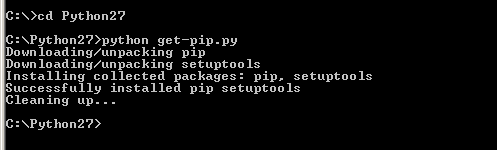3.Install Coursera-dl script

• In the command line change directory to pip (C:\Python27\Scripts for my case)
• execute pip install coursera-dl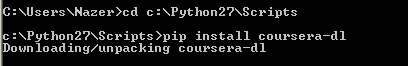4.Now you are ready to download materials! For this you need the class name identificator from the browser URL.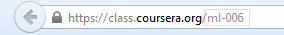Provide your user name and password, directory to download and class name identificator as the parameters into coursera-dl script. Don’t forget, that you have to accept the honor code of the class before using this script (happens the very first time you go to the class page).